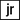# The Revised Julian Calendar

Project originally posted on reddit.com/r/dailyprogrammer

# Background

The Revised Julian Calendar is a calendar system very similar to the familiar Gregorian Calendar, but slightly more accurate in terms of average year length. The Revised Julian Calendar has a leap day on Feb 29th of leap years as follows:

• Years that are evenly divisible by 4 are leap years.
• Exception: Years that are evenly divisible by 100 are not leap years.
• Exception to the exception: Years for which the remainder when divided by 900 is either 200 or 600 are leap years.

For instance, 2000 is an exception to the exception: the remainder when dividing 2000 by 900 is 200. So 2000 is a leap year in the Revised Julian Calendar.

# Challenge

Given two positive year numbers (with the second one greater than or equal to the first), find out how many leap days (Feb 29ths) appear between Jan 1 of the first year, and Jan 1 of the second year in the Revised Julian Calendar. This is equivalent to asking how many leap years there are in the interval between the two years, including the first but excluding the second.

``````leaps(2016, 2017) =&gt; 1
leaps(2019, 2020) =&gt; 0
leaps(1900, 1901) =&gt; 0
leaps(2000, 2001) =&gt; 1
leaps(2800, 2801) =&gt; 0
leaps(123456, 123456) =&gt; 0
leaps(1234, 5678) =&gt; 1077
leaps(123456, 7891011) =&gt; 1881475``````

For this challenge, you must handle very large years efficiently, much faster than checking each year in the range.

``leaps(123456789101112, 1314151617181920) =&gt; 288412747246240``

## Solution

``````function leaps(year1, year2) {
let count = 0;
for (let i = year1; i < year2; i++) {
const rule1 = i % 4 ? false : true;
const rule2 = i % 100 ? false : true;
const rule3 = i % 900 === 200 || i % 900 === 600 ? true : false;

if (rule1) {
if (rule2) {
if (rule3) {
count++;
continue;
}
continue;
}
count++;
}
}
return count;
}``````

## Tests

``````describe('leap years', () => {
it('should find the leap years', () => {
expect(leaps(2016, 2017)).toEqual(1);
expect(leaps(2019, 2020)).toEqual(0);
expect(leaps(1900, 1901)).toEqual(0);
expect(leaps(2000, 2001)).toEqual(1);
expect(leaps(2800, 2801)).toEqual(0);
expect(leaps(123456, 123456)).toEqual(0);
expect(leaps(1234, 5678)).toEqual(1077);
expect(leaps(123456, 7891011)).toEqual(1881475);
});
});``````